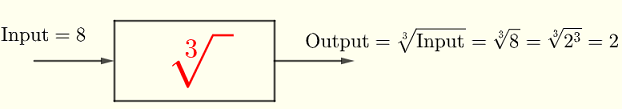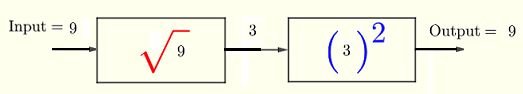# What Are Radicals in Math?

Radicals in maths are defined with examples and detailed solutions. Questions with their solutions are also presented. 

## Power of $n$

Let the following power of $2$ (or exponent ) operation be represented by the diagram below:More examples of inputs and outputs of the power $2$ operation
Input = $4$   ,   Output = $4^2 = 4 \times 4 = 16$
Input = $10$   ,   Output = $10^2 = 10 \times 10 = 100$
The diagram below represents the power $3$ (or exponent ) operation.More examples of inputs and outputs of the power $3$ operation
Input = $3$   ,   Output = $3^3 = 3 \times 3 \times 3 = 27$
Input = $1$   ,   Output = $1^ 3 = 1 \times 1 \times 1 = 1^3$
Input = $4$   ,   Output = $4^3 = 4 \times 4 \times 4 = 64$
General Power of $n$

$a^n = a \times a \times a .... \times a$ , n times

### Radical with index 2 (or Square Root) is the inverse of power 2

Let us now represent the inverse operation of the power $2$ operation as shown in the diagram below.
We interchange the input $3$ and output $9$ of the power 2 operation, shown above, to become respectively the output $3$ and input $9$ of the inverse operation as shown below.and we write: $\sqrt[\color\red{\Large 2}]{ 9 } = 3 \text{ because } 9 = 3^{\color\red{2}}$
More examples of inputs and outputs
Input = $16$ , Output = $\sqrt{16} = 4$     because   $4^2 = 16$
Input = $25$ , Output = $\sqrt{25} = 5$     because  $5^2 = 25$
The symbol $\sqrt{ }$ is called the radical and $2$ is the index of the radical. The number (or expression) inside tha radical is called radicand . This operation is called the square root.NOTE By convention, the symbol for the radicals with index 2 (or square root) is written without the index $2$ as $\sqrt{\;\;}$.

### Radicals with index 3 (or Cube Root) is the inverse of power 3

The inverse operation of the power $3$ operation as shown in the dagram below is called the cube root.and we write: $\sqrt[\color\red{\Large 3}]{ 8 } = 2 \text{ because } 8 = 2^{\color\red{3}}$
More examples of inputs and outputs
Input = $27$ , Output = $\sqrt{27} = 3$ because $3^3 = 27$
Input = $125$ , Output = $\sqrt{125} = 5$ because $5^3 = 125$

### In general Radicals with index $n$ (or $n^{th}$ Root) is the inverse of power $n$

We now generalize and define the radicals with index $n$ where $n$ is a whole number.

If $y = a^n$ , then $\sqrt[n]{y} = a$    (I)

More Examples
$y = 3^5 = 243$ , therefore $\sqrt{243} = 3$
$y = 10^6 = 1000000$ , therefore $\sqrt{1000000} = 10$
$y = (-2)^3 = -8$ , therefore $\sqrt{-8} = -2$
$y = 1^{20} = 1$ , therefore $\sqrt{1} = 1$     in general $\sqrt[n]{1} = 1$ for any whole number $n$
$y = 0^9 = 0$ , therefore $\sqrt{0} = 0$     in general $\sqrt[n]{0} = 0$ for any whole number $n$
NOTE The relationships in (I) are not valid if $n$ is an EVEN integer and $y$ is a NEGATIVE number.
a) $\sqrt{-16}$ is UNDEFINED in the real numbers because no real number $x$ exists such that $x^2 = -16$ since the square of a real number is always positive or zero.
b) $\sqrt{-1}$ is UNDEFINED in the real numbers because no real number $x$ exists such that $x^4 = - 1$ for the same reason as above.

## The Power and the Corresponding Radical Undo Each Other

We say that radicals and corresponding (same index) power operations undo each other. If we apply the two operations successively, the output is equal to the input because the two operations are inverse of each other.
The radicals with index 2 (or square root) undoes the power $2$ as shown in the diagram below the output is equal to the input.The above may be written as $\sqrt{3^2} = 3$
The power $2$ undoes the radicals with index 2 (or square root) as shown in the diagram below the output is equal to the input.The above may be written as $(\sqrt{9})^2 = 9$
Note that the radical with index $2$ is written as $\sqrt{\;\;}$ (i.e without index)

More examples: $(\sqrt {12})^2 = 12$ , $\sqrt {8^2} = 8$
The radicals with index 3 (or cube root) and power $3$ undo each other.
Examples: $(\sqrt{5})^3 = 5$ , $(\sqrt{10})^3 = 10$
In general and for $a \ge 0$, we can write

$\sqrt[n]{a^n} = a$ , $(\sqrt[n]{a}\;)^n = a$    (II)

## Questions (with solutions given below)

DO NOT use the calculator to answer the follwoing questions

Part 1 - Given the following:
$2^6 = 64$ , $\; 3^5 = 243$ , $\; 5^3 = 125$, $\; 0^7 = 0$, $\; 1^{20} = 1$, $\; 2^9 = 512$, $\; 5^5 = 3125$, $\; 10^5 = 100000$ , $\; 0.1^3 = 0.001$
find the values of the following:
$\sqrt{512}$ , $\; \sqrt{3125}$ , $\; \sqrt{243}$ , $\; \sqrt{64}$ , $\; \sqrt{0.001}$ , $\; \sqrt{1}$ , $\; \sqrt{100000}$ , $\; \sqrt{0}$ , $\; \sqrt{125}$

Part 2 - Given the following:
$\sqrt{64} = 8$ , $\; \sqrt{7776} = 6$ , $\; \sqrt{1000} = 10$ , $\; \sqrt{128} = 2$ , $\; \sqrt{0.0000001} = 0.1$ , $\; \sqrt{10000} = 100$ , $\; \sqrt{20736} = 12$ , $\; \sqrt{512} = 2$
find the values of the following:
$2^7$ , $\; 0.1^7$ , $\; 6^5$ , $\; 8^2$ , $\; 2^9$ , $\; 12^4$ , $\; 10^3$ $\; 100^2$

Part 3 - Simplify the following:
$\sqrt{5^2}$ , $\; (\sqrt{3})^5$ , $\; \sqrt{10^3}$ , $\; (\sqrt{128})^7$

## Solutions to the Above Questions

Part 1
Given:    $2^6 = 64$ , $\; 3^5 = 243$ , $\; 5^3 = 125$, $\; 0^7 = 0$, $\; 1^{20} = 1$, $\; 2^{9} = 512$ , $\; 5^5 = 3125$, $\; 10^5 = 100000$ , $\; 0.1^3 = 0.001$

$\sqrt{512} = 9$ because we are given $\; 2^9 = 512$ (remember we do not write index of radical when it is equal to $2$ ).

$\sqrt{3125} = 5$ because   $5^5 = 3125$

$\sqrt{243} = 3$ because   $3^5 = 243$

$\sqrt{64} = 2$ because   $2^6 = 64$

$\sqrt{0.001} = 0.1$ because   $0.1^3 = 0.001$

$\sqrt{1} = 1$ because   $1^{20} = 1$ and note that for any $n \gt 0$ , $\sqrt[n]{1} = 1$

$\sqrt{100000} = 10$ because   $10^5 = 100000$

$\sqrt{0} = 0$ because   $0^7 = 0$ and note that for any $n \gt 0$ , $\sqrt[n]{0} = 0$

$\sqrt{125} = 3$ because   $5^3 = 125$

Part 2
Given:    $\sqrt{64} = 8$ , $\; \sqrt{7776} = 6$ , $\; \sqrt{1000} = 10$ , $\; \sqrt{128} = 2$ , $\; \sqrt{0.0000001} = 0.1$ , $\; \sqrt{10000} = 100$ , $\; \sqrt{20736} = 12$ , $\; \sqrt{512} = 2$

$2^7 = 128$ because we are given $\sqrt{128} = 2$

$0.1^7 = 0.0000001$ because   $\sqrt{0.0000001} = 0.1$

$6^5 = 7776$ because   $\sqrt{7776} = 6$

$8^2 = 64$ because   $\sqrt{64} = 8$

$2^9 = 512$ because   $\sqrt{512} = 2$

$12^4 = 20736$ because   $\sqrt{20736} = 12$

$10^3 = 1000$ because   $\sqrt{1000} = 10$

$100^2 = 10000$ because   $\sqrt{10000} = 100$

Part 3
$\sqrt{5^2} = 5$ because square root (radical with index 2) and power of $2$ undo each other

$(\sqrt{3})^5 = 3$ because power of $5$ and and radical of index $5$ undo each other

$\sqrt{10^3}$ because cube root (radical with index 3) and power of $3$ undo each other

$(\sqrt{128})^7 = 128$ because power of $7$ and and radical of index $7$ undo each other